Example Questions

1 2 3 4 6 Next →

Example Question #81 : Circles

What is the area, in square feet, of a circle with a circumference of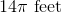?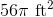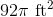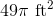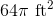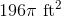Explanation:

In order to find the area of a circle with a known circumference, first solve for the radius of the circle.

We know the circumference of a circle is equivalent to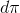, where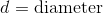.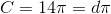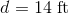The radius of a circle is equal to half the diameter.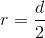Therefore: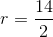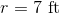The area of a circle is given by the equation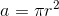. Use the radius to solve for the area.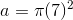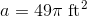The area of a circle with circumference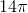is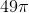square feet.

Example Question #81 : Plane Geometry

A square has a perimeter of 48 inches. What is the area, in square inches, of the largest circle that will fit entirely inside the square?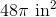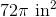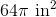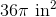Explanation:

A perimeter of a square is equal to the sum of the four equal sides: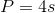Therefore, the length of one side of this square is 12: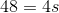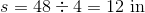We know the largest circle that can fit entirely inside the square will have a maximum diameter of 12 (the length of one side of the square).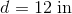To find the area of this circle, we must find the radius by dividing the diameter by 2: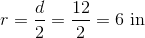The radius of the circle is 6. Using the formula for area, we find: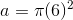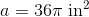The area of the largest circle that will fit inside a square with a perimeter of 48 inches issquare inches.

In the following diagram, the radius is given. What is area of the shaded region?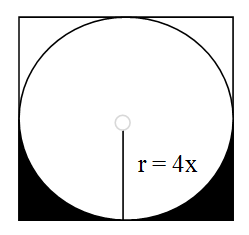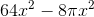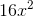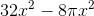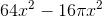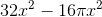Explanation:

This question asks you to apply the concept of area in finding both the area of a circle and square. Since the cirlce is inscribed in the square, we know that its diameter (two times the radius) is the same length as one side of the square. Since we are given the radius,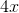, we can find the area of both the circle and square.

Square: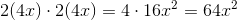This gives us the area for the entire square.

The bottom half of the square has area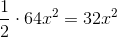.

Now that we have this value, we must find the area that the circle occupies. The area of a circle is given by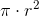.

So the area of this circle will be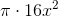.

The bottom half of the circle has half that area: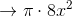Now that we have both our values, we can subtract the bottom half of the circle from the bottom half of the square to give us the shaded region: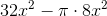1 2 3 4 6 Next →